# How Do You Calculate Square Footage?

### Alcove Team·2/24/22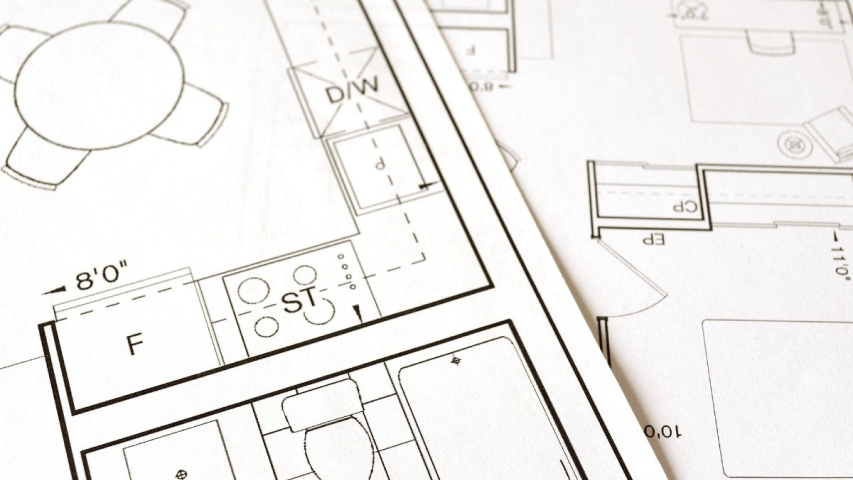## Here is how to calculate square footage:

A square foot is a unit of area which is used in real estate for measuring property. It helps the client to decide the size of his house/room easily according to his pocket.

Square footage is calculated by using a mathematical formula. This method of property measuring is used primarily in the United States, Asia (Pakistan, India, Bangladesh, Singapore, etc.), and partially in Canada. It is a non-SI unit of area.

## What is square footage?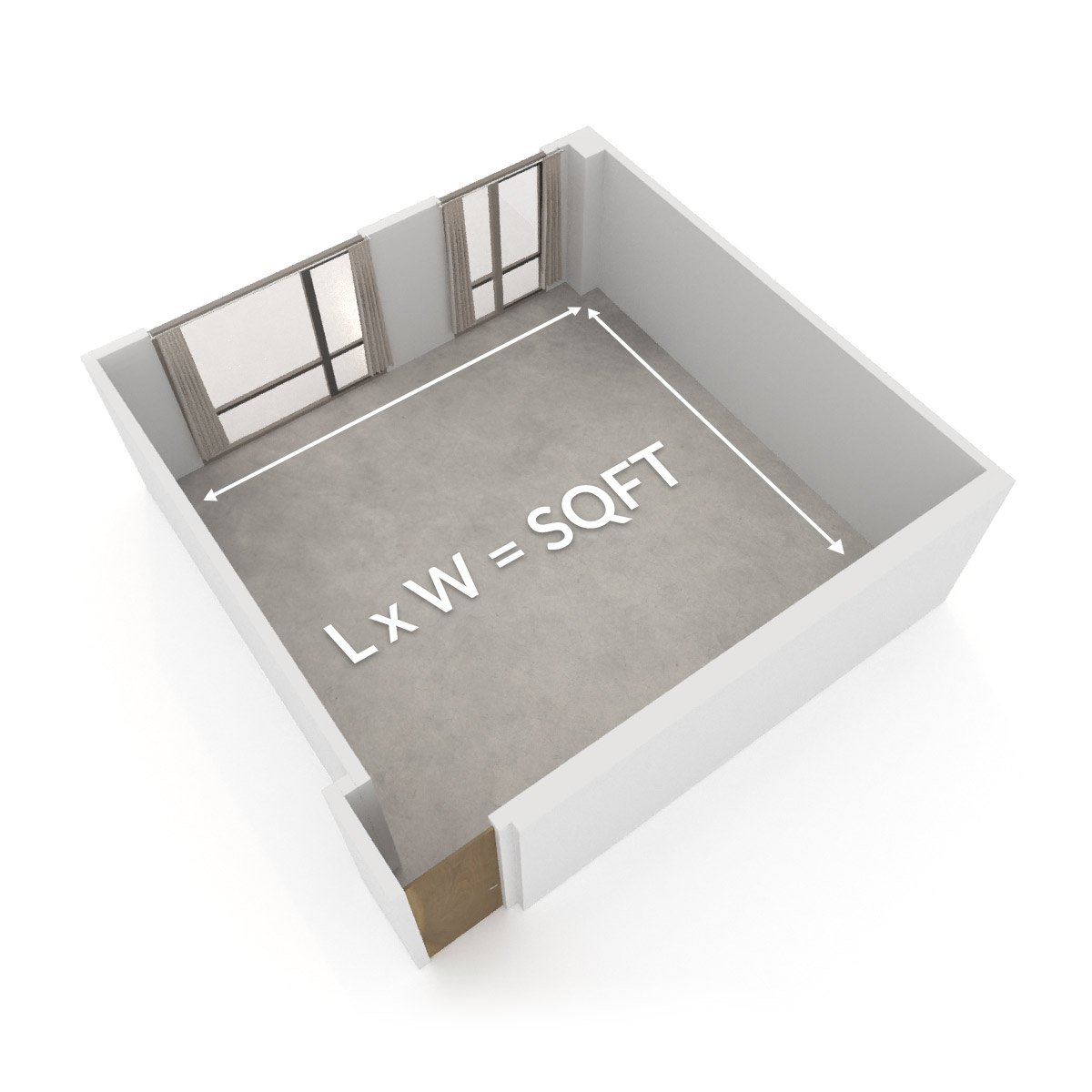The measurement of any two-dimensional place is square foot/ footage. Any closed two-dimensional space, like a square, rectangle, or circle, can have square footage. But the formula or equation to calculate square footage will differ for every shape.

## Formulas to calculate square footage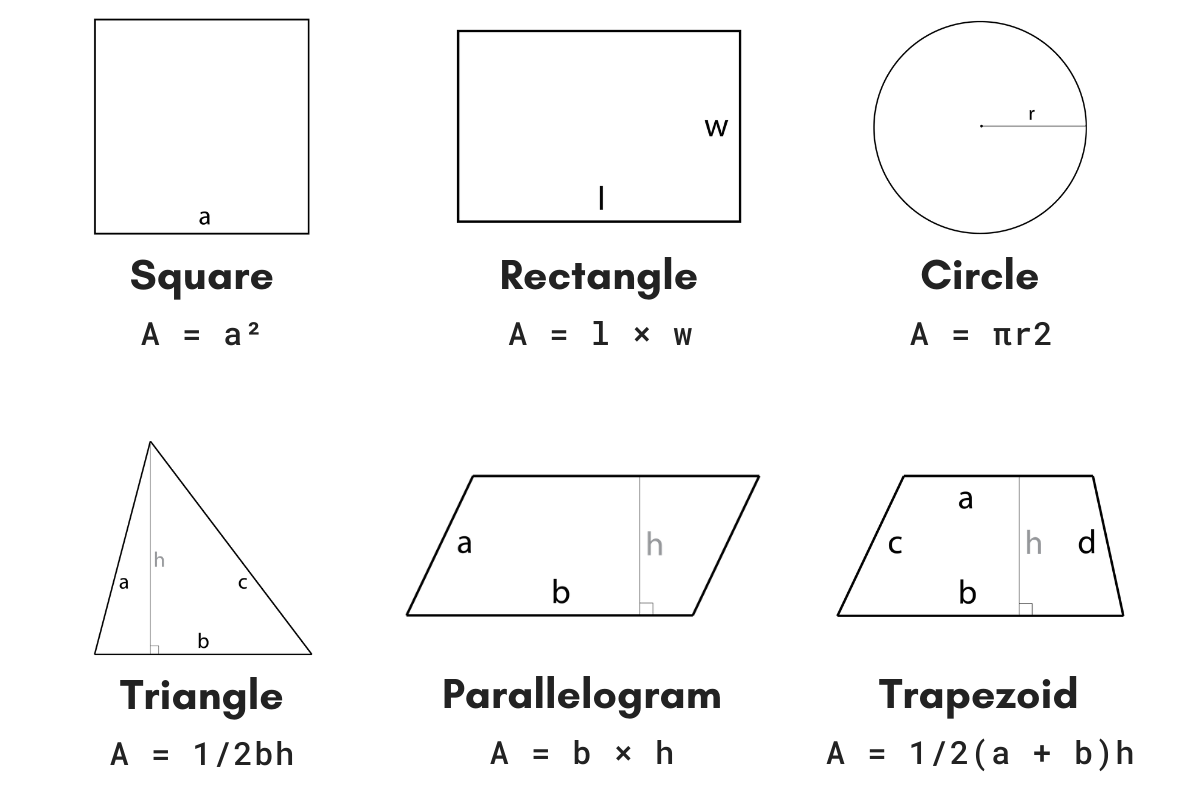Formulas to calculate square footage will depend upon the shape of which we are calculating the area or square footage. Following are some formulas:

• Area of square = length × length (square has all four equal sides).

• Area of rectangle = length × width.

• Area of circle = 3.14 × radius × radius (a line segment from the center of the circle to its boundary is called the radius).

• Area of triangle = 1/2 base × height.

## Example of calculating square footage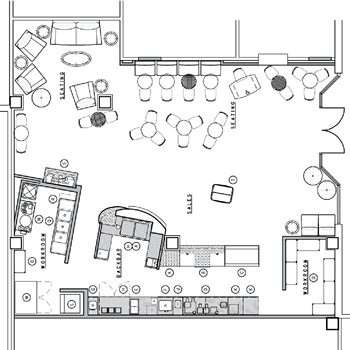Let us take an example of a coffee shop and calculate its square footage to understand its concept. If the shop is of squared shape and has each side equal to 30 feet, we will multiply any two sides of the square to get square footage. So, the area will be equal to 900 square feet. By using the formula

Area of square = length × length.

Area of square = 30 feet × 30 feet.

Area of square = 900 square feet.

But if the shape of the coffee shop will change, it will also affect the area or square footage of the shop. The new area will be calculated with the help of the formulas as mentioned earlier according to the available shape.

## Steps to calculate square footage of a house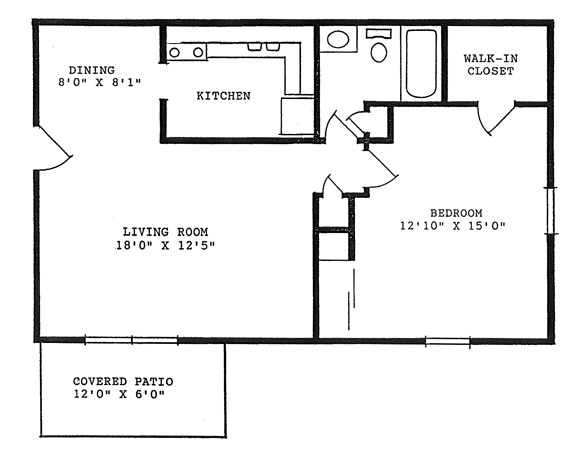If a house is big enough to calculate square footage, you should divide the house into small parts. For a room, the process would become more manageable.

Things that a person need to calculate square footage are:

• Long measuring tape

• Pencil

• Graph paper (optional)

Consider one box on the graph equals one foot. Start moving around the house with all the things mentioned above and measure each house floor. Now the graph paper will have a complete picture of the house. By using this, use the suitable formula to calculate square footage.

Note that for measuring the area of a home, more than one formula can be used according to the shapes in which the house is divided.

## Steps to calculate square footage of a room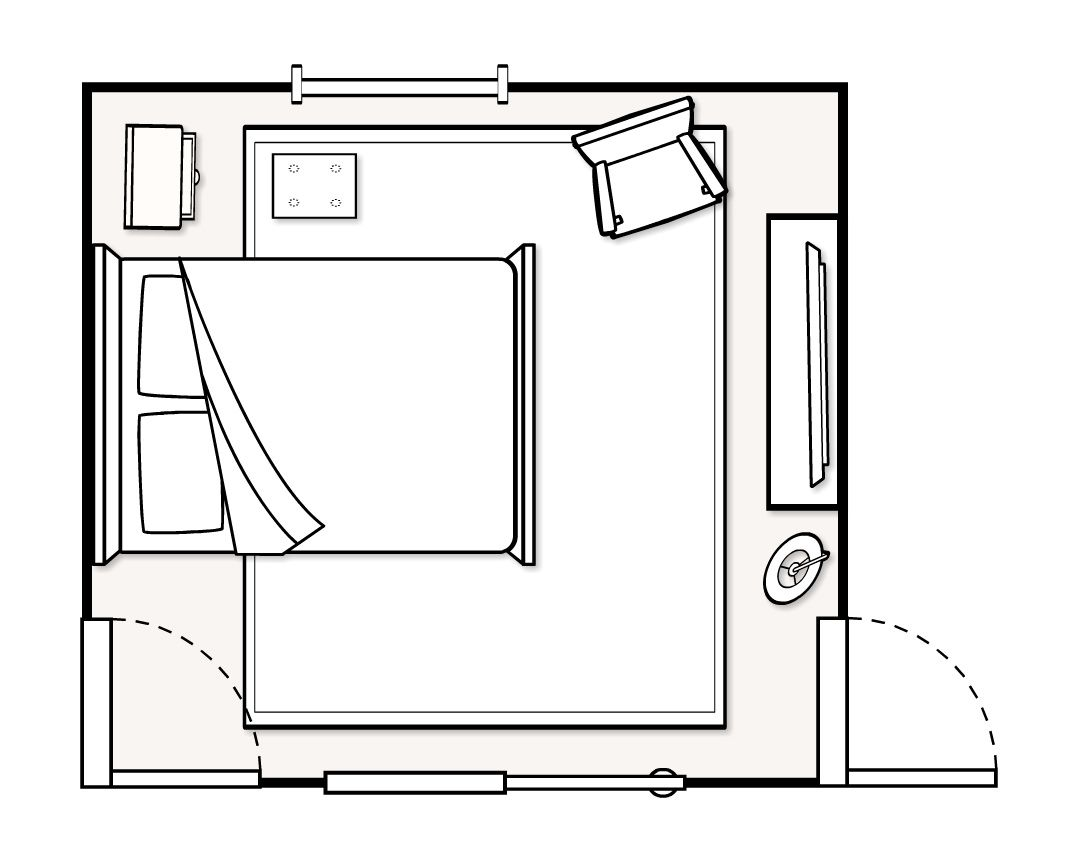A room is just like a rectangle or a square that has four sides. To calculate the square footage of a room, you just have to use the formula of area of a rectangle or square as mentioned above.

And if your room has a closet, then consider the closet as a rectangular extension itself. This way it will be easier to calculate the area of tour rooms.

## Apps to calculate square footage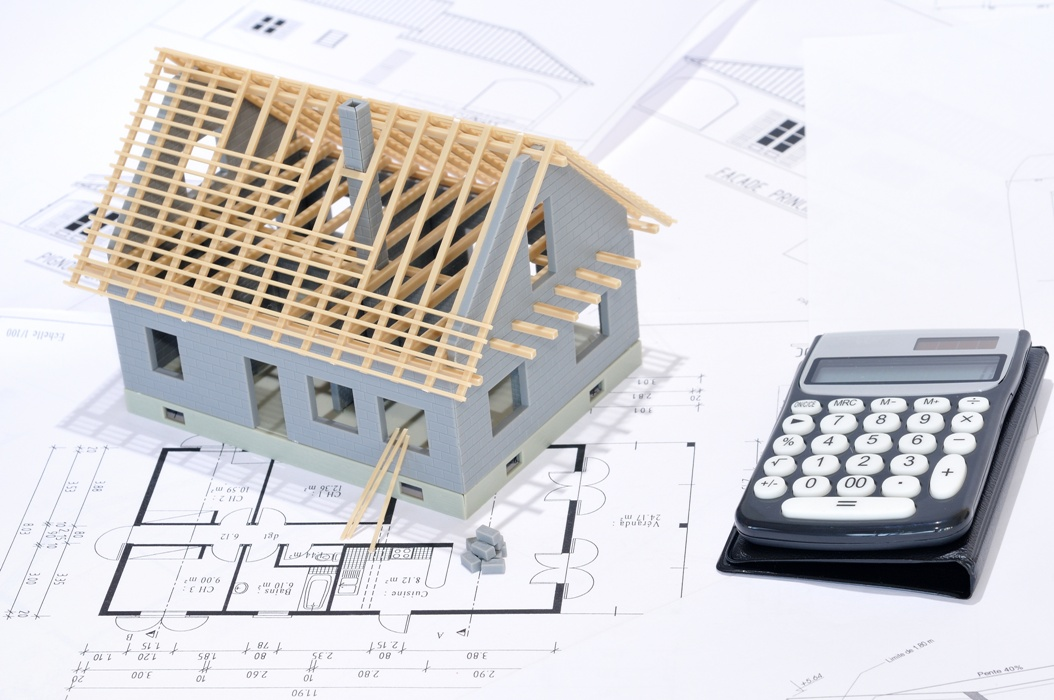Developers have developed apps to calculate the square footage of any closed surface. The measure app in iPhone uses AR (augmented reality) technology to measure the area of any object. Some of the apps for Android and iOS users to measure square footage are listed below:

## Importance of square footage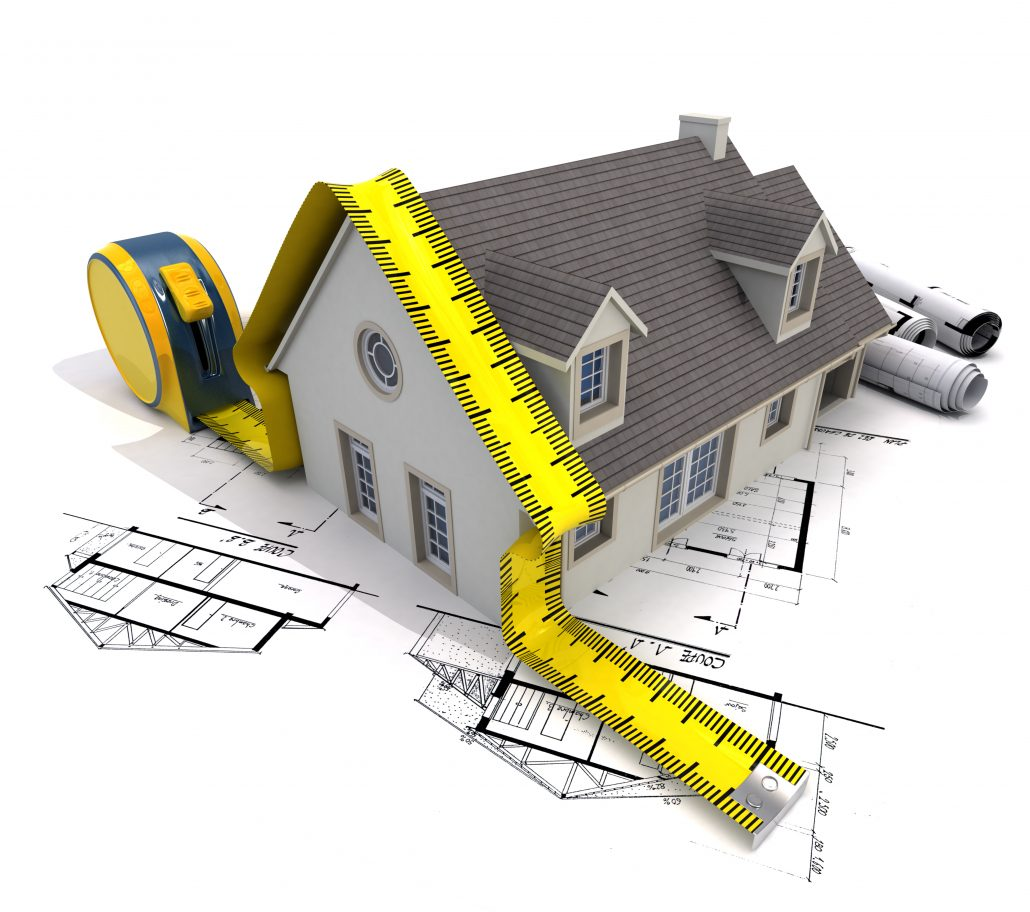• To calculate the cost of the property to be sold or bought.

• To renovate or decorate your place.

• It will affect the lifestyle options you have.